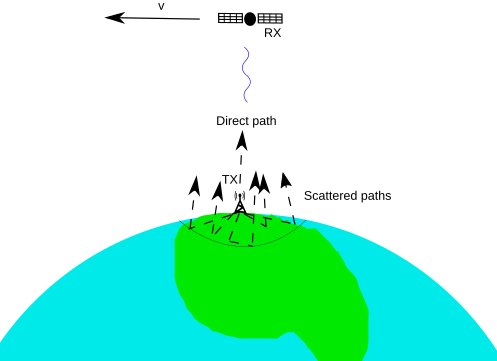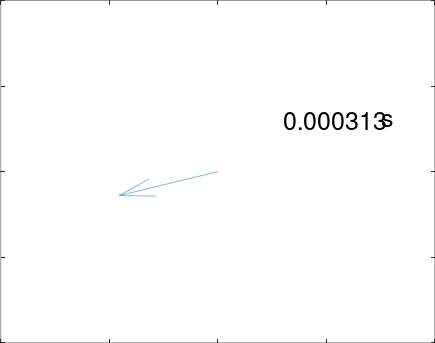## May – Signal fading in a satellite receiver and the coherence timeIn this blog post, we study a faded signal in an omnidirectional satellite receiver sent by an earth transmitter. We consider that the transmitter (TX) is sending a constant QAM symbol, i.e., the TX baseband signal is given by(1)

for someand. The passband signal experiences fading due to terrestrial obstacles. We use a model wherescatterer objects are Poisson distributed inside a circular area of diameterm. The phases of the scattered signals are independently uniformly random. Initially, the satellite, which is moving at its orbital speed, is in the zenith w.r.t. the earth transmitter. The receiver's (RX) baseband signal is a linear combination of the down-conversions of the scattered, Doppler shifted and attenuated passband signal components, and it is given by(2)

whereis the path-loss function at distancefrom the scatterer,is the direct-path distance to the transmitter,is the parameter that determines the energy ratio of the direct-path (LOS) component and the scattered paths, andis the propagation delays restricted by the speed of light, which is dependent on timeas the satellite moves, andis the carrier frequency.

Given this model, we are interested in the time scale during whichvaries, i.e., the coherence time. The coherence time depends on the Doppler shift, and the orbital speed of the satellite is given by(3)

wherems, and the denominator is the sum of the altitude of the satellite and the radius of the earth, respectively. This is fast: aroundkm/s atkm. However, Doppler shift is only caused by the velocity component that is directed towards the transmitter. The Doppler shift is zero when the satellite is in the zenith and grows at lower elevation angles. In our model, the scatterers were distributed in an area of diameterm below the satellite, and the maximum Doppler shift initially isHz for, hence the Doppler spreadHz. The coherence time is given bys(4)

The satellite moves aboutmeters during this period.

The following figures illustrate the RX baseband signal changing in time while the satellite moves. As expected, the variance in the signal strength is larger in the Rayleigh faded case.Here is the Octave code:

function signals = satellite_baseband_simulation()
close all;
clear all;
clear imwrite;

h = 600*1000; #Altitude of satellites.
K = 0; #Rician parameter.
t = 0; #Initial time.
[refs bbsignals] = scatteredsignals(K);  #Random baseband signals ”bbsignals' and the scatterer-obstacle's locations in 'refs'.
signal = RXbaseband(refs, bbsignals);
N = 200;
history =[];
filename = 'basebandgif.gif';
if(exist(filename))
delete(filename);
end

for iii = 1 : N
##Observe the progress.
if(mod(iii,10) == 0)
iii
end
refs = rotateearth(refs); #Rotate Earth.
signal = RXbaseband(refs, bbsignals); #New received baseband signal
history = [history [real(signal); imag(signal)]]; #History of the signals.
##Write GIF.
quiver(0,0,real(signal), imag(signal));
hold on;
plot(history(1,:), history(2,:))
axis([[-0.1, 0.1], [-0.1, 0.1]]);
t = t + 1/(4*8*100); #Time hop. t = 1/(8*300) is the initial coherence time of the example.
text(0.03, 0.03, mat2str(t, 3), 'fontsize',25);
text(0.075, 0.03, 's', 'fontsize',25);
frame = getframe();
imwrite(frame.cdata, filename,'gif','writemode','append','DelayTime',0.05, 'Compression','lzw')
hold off;
end
end

##Returns a table of 101 (1 LOS signal and 100 signals from the scattered paths) randomly phased complex baseband signal symbols each corresponding to one of a obstacle location given in 'refs'. 'K' is the Rician parameter.
function [refs, signals] = scatteredsignals(K)
##First, generate the Poisson distributed random obstacle locations.
refs = [0; 0]; #LOS component.
yMin = 1-0.0000000001; yMax = 1;
xMin = -pi; xMax = pi;
xDelta = xMax - xMin; yDelta = yMax - yMin; #Rectangle dimensions
numbPoints =100;    #Number of points.
x = xDelta*(rand(numbPoints,1)) + xMin;    #Pick points from uniform distribution
y = yDelta*(rand(numbPoints,1)) + yMin;
refs = [refs [pi/2-asin(y)' ; x'] ]; #Map referencepoints to spherical coordinates
A = 30000; #Amplitude.
signals = [A*sqrt(K/(K+1)).*exp(-rand(1,1).*i*2*pi)];
signals = [signals A/(sqrt(100)*sqrt(1+K)).*exp(-rand(1,length(refs(1,:))-1).*i*2*pi)];
end

function bb = RXbaseband(refs, signals)
R = 6378*1000; #Radius of Earth in m.
h = 600*1000; #Altitude of the satellite.
d = @(gamma) sqrt((cos(gamma).*(R+h)-R).^2+(sin(gamma).*(R+h)).^2); ##Distance to the satellites in m.
c = 299792458; ##Speed of light.
if(!isempty(refs))
a = 1./d(refs(1,:));
tau  = d(refs(1,:))/c;
else
a = 0;
tau = 0;
end
fm = 12*10^9; ##Modulation frequency.
ab = a.*exp(-i*2*pi*tau.*fm); ##Received individual signals.
bb = sum(ab.*signals); ##Aggregate received signal.
end

##Rotates the positions in 'refs' as the satellite "moves". In this model, the satellite stays still at (0,0) but the Earth moves.
function refs = rotateearth(refs)
t = 1/(4*8*100); #Time hop in seconds.
h= 600*1000; #Altitude of the satellite.
R = 6378*1000; #Radius of Earth.
GM = 3.986*10^14; #Gravitational constant.
orbitalspeed = sqrt(GM/(h + R)); #Satellite speed in m/s.
angularspeed = orbitalspeed/(h + R); #Angular speed of the satellite.
rotation = angularspeed*t; #Rotation of Earth.
eucpos  = pol2euc(refs); #Transform the polar coordinates to euclidean coordinates.
newpos = [[1 0 0]; [0 cos(rotation) -sin(rotation)]; [0 sin(rotation) cos(rotation)]]*eucpos; #Rotation about x-axis.
refs = euc2pol(newpos); #Back to the polaroordinates.
end

function p  = euc2pol(e)
R = 6378*1000; #Radius of Earth.
p = [acos(e(3,:)./R); (e(2,:) >= 0).*atan2(e(2,:),e(1,:)) + ...
(e(2,:) < 0).*(atan2(e(2,:),e(1,:)) + 2*pi)]; #Polar coordinates from the given Euclidean coordinates in 'e'..
end

function e = pol2euc(p)